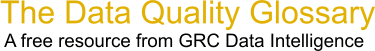Choose Index below for a list of all words and phrases defined in this glossary.

# Process Capability Index (Cp, Cpk)

index | Index

## Process Capability Index (Cp, Cpk) - definitions

Process Capability Index - The value of the tolerance specified for the characteristic divided by the process capability. The several types of process capability indexes include the widely used Cpk and Cp.

[Category=Quality ]

Source: American Society for Quality, 13 October 2010 09:55:55, http://www.asq.org/glossary/These advertisers support this free service

Cp - Process Capability Index_: a measure of the ability of a process to produce consistent results - the ratio between the permissible spread and the actual spread of a process. Permissible spread is the difference between the upper and lower specific limits of acceptibility (a.k.a. total tolerance); actual spread is defined, arbitrarily, as the difference between upper and lower 3 x sigma deviations from the mean value (representing 99.7% of the normal distribution). As a formula, Cp = (USL-LSL)/(6 x sigma). Note this takes no account of how well the output is centered on the target (nominal) value. For that see Cpk Index.

You can think of the process capability Index_ Cp in 3 ways:

```   1. Cp measures the capability of a process to meet its specification limits. It is the ratio between the required and actual variability.
2. More mathematically, the Cp is the ratio of the Spec difference (upper - lower) divided by 6-sigma, which is the spread of a normal curve. Minitab gives the following explanation: 'Capability statistics are basically a ratio between the allowable process spread (the width of the specification limits) and the actual process spread (6s)'
3. Graphically, think of positioning a normal curve centered between the specs. Now look at the tail areas that exceeds the specs. The smaller the area, the larger the Cp. In this sense it is equivalent to looking at the popular PPM measure (parts-per-million) which gives the area of the normal curve that exceeds the specs.
```

[Category=Data Quality ]

Source: iSixSigma, 12 January 2011 10:27:10, http://www.isixsigma.com/index.php?option=com_glossaryCpk - Process Capability Index_ ('equivalent') taking account of off-centredness: effectively the Cp for a centered process producing a similar level of defects - the ratio between permissible deviation, measured from the mean value to the nearest specific limit of acceptability, and the actual one-sided 3 x sigma spread of the process. As a formula, Cpk = either (USL-Mean)/(3 x sigma) or (Mean-LSL)/(3 x sigma) whichever is the smaller (i.e. depending on whether the shift is up or down). Note this ignores the vanishingly small probability of defects at the opposite end of the tolerance range. Cpk of at least 1.33 is desired.

Capability analysis indice.

[Category=Data Quality ]

Source: iSixSigma, 12 January 2011 10:27:53, http://www.isixsigma.com/index.php?option=com_glossaryProcess Capability Index_ - Process Capability Index_ is used to find out how well the process is centered within the specification limits.It is denoted by Cpk.

```   Cpk = Cp(1-K)
```

Where,

```   Cp = Process Capability
```
```   K = 2(Design Target - Process Average) / (USL - LSL)
```

Design target is the actual specification targetted without +/- allowance.

[Category=Data Quality ]

Source: iSixSigma, 14 February 2011 08:55:36, http://www.isixsigma.com/index.php?option=com_glossaryData Quality Glossary.  A free resource from GRC Data Intelligence. For comments, questions or feedback: dqglossary@grcdi.nl